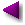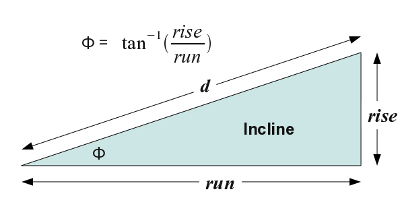Home | Science | * Gravitation Equations | * Gravitation Description and Equations 02. Force & Weight 03. Power, Energy & Gravity 04. Ballistics 05. Orbital Mechanics 06. The PendulumShare This Page
Power, Energy & Gravity

Dynamics of gravitational equations

(double-click any word to see its definition)

Note: This is a multi-page article.
To navigate, use the drop-down lists and arrow keys at the top and bottom of each page.

Power

Power, with units of watts, is the rate at which work is performed or energy is expended. In a mechanical problem, power is the time derivative of energy or work. In the present context, power is the instantaneous product of force times distance divided by time:

(1) $\displaystyle p = \frac{f\ d}{t}$
Where:
• p = Power, watts.
• f = Force, newtons
• d = Displacement, meters
• t = Time, seconds

Because $\rm{\frac{displacement}{time}}$ equals velocity, there is a more useful, alternate form of the above equation:

(2) $\displaystyle p = f\ v$

Where v = velocity, with units of meters per second.

I ask my readers to notice about equation (2) that, for power to be expended, there must be both force and velocity, and the force must be applied in the direction of the velocity. A static force without velocity does not require power to maintain itself, and velocity (including rotational velocity) without force also does not require power.

Again, for power to be expended:
• Force and velocity must both be present, and
• The force must be applied in the direction of the velocity.

This definition may seem excessively strict and detailed, but consider a spinning top — it has both force (a centripetal force at right angles to its velocity) and rotational velocity, but no power is required to maintain the velocity (the top will only slow down if friction is present). The reason is that the force's direction is at right angles to the velocity's direction.

Energy

Energy, with units of joules (or watt-seconds), is power multiplied by time. This means the expenditure of 100 watts for 30 minutes represents an energy expenditure of (100 x 30 x 60) 180,000 joules.

Remember that energy is always conserved. If energy is expended lifting a weight, the energy must have had some prior form (even sometimes as mass). The act of lifting the mass represents an example of energy conversion (potential to kinetic energy), and after the mass has been lifted, the energy expended in lifting it remains as (a) gravitational potential energy and (b) a small amount of additional mass.

Lifting a Mass

Remember the discussion above — lifting a mass against gravity requires power, and power applied over time requires energy. The difference between power and energy is a Calculus idea (energy is the time integral of power), but it's not necessary to understand much Calculus to grasp this idea. (For those who want to learn Calculus, click here.)

We have decided to lift the previous example's ten-kilogram mass from the table to a high shelf, a distance of three meters. We will compute the required energy first, then discuss power later, for a reason that will become obvious.

For a given mass m, a height h over which the mass is to be raised, and a gravitational acceleration g, we compute an energy quantity called work, or force times distance, with units of joules:

(3) $W = mgh$

Where:

• W = Mechanical work, or force times distance, energy with units of joules
• m = Mass, kilograms
• g = Little-g, discussed earlier
• h = height over which the mass is lifted, meters

To lift a ten-kilogram mass three meters against the force of gravity, we must expend this much energy:

(4) $W = mgh = 10 \times 9.80665 \times 3 = 294.1995\ \text{joules}$

Let's round this off to 294 joules for the next discussion. Okay, we have the total energy required, but how much power is needed? Remember that power is equal to force times velocity (equation (2) above), and energy is power multiplied by time. This means all the solutions below will work:

• A power of 294 watts for one second.
• A power of 29.4 watts for ten seconds.
• A power of 2.94 watts for 100 seconds.

Remember this relationship between power and energy — a small amount of power expended over a long time can accomplish the same task as a large amount of power over a short time. But in all cases, the energy required is the same.

Potential and Kinetic Energy

In simple mechanical systems, energy has two primary forms — potential and kinetic. Potential energy is the energy of position or state — a coiled spring, gas under pressure, a book on a high shelf. Kinetic energy is energy of motion — an arrow in flight, a weight being lifted, a satellite in orbit. Many mechanical problems turn out to be descriptions of energy conversions — potential to kinetic and back again. And remember — regardless of the details, energy is always conserved — it may be changed in form, but it is never created or destroyed.

Here is a detailed description of the above mass-lift problem expressed in terms of energy:

• Let's say there is a coiled spring beneath the ten-kilogram mass, and the spring stores mechanical energy equal to 294 joules.
• Before time zero, the spring is kept from releasing its tension, its energy. The spring's stored potential energy causes the spring's mass to increase slightly, according to this Special Relativity principle:
(5) $m = \frac{E}{c^2}$

Where:

• m = mass, kilograms
• E = Energy, joules
• c = The speed of light, defined as 299,792,458 m/s.

Because of its potential energy of 294 joules, the coiled spring gains this much mass:

(6) $m = \frac{E}{c^2} = \frac{294}{299792458^2} = 3.27 \times 10^{-15}\ \text{kilograms}$

This calculation shows that the spring has an energy equivalent mass increase of just over three femtograms, about the mass of five E. coli bacteria.

• At time zero, the spring is released and expends its 294 joules of energy raising the ten-kilogram mass three meters.

The spring's potential energy becomes kinetic energy, the energy of motion.

The time this motion takes doesn't matter — as explained above it could be a fraction of a second, or it could be many minutes. The only issue is whether a total mechanical energy of 294 joules is expended.

• The spring reaches its full height and the mass is deposited on a high shelf.

What had been mechanical tension in the spring became kinetic energy during the lift, and what had been kinetic energy is now gravitational potential energy in the mass sitting on the shelf. The mass now has the energy equivalent mass increase — 3.27 femtograms — that was lost by the spring.

Climbing a Hill

A car must climb a grade at a certain speed. We will use the methods described above to determine how much horsepower is needed to achieve a specified climb rate. Here are the details:Figure 2: Slope Diagram
• Vehicle mass m: 3000 kilograms
• Hill slope (rise/run): 0.12.
• Hill angle φ = $\displaystyle tan^{-1}(rise/run)$ = 6.84°
• Desired velocity v: 15 m/s (54 kph).

A nomenclature notice. Hill slopes are sometimes expressed as grade percentages, where the value is equal to:

(7) $\displaystyle grade\ \% = 100 \frac{rise}{run}$

Using this nomenclature, our hill slope of 0.12 would be described as a 12% grade. To convert such a grade into an angle, compute:

(8) $\displaystyle \phi = tan^{-1} \left(\frac{grade}{100} \right)$.

When climbing a grade, we experience less gravitational force than in a vertical lift. The force equation for this case is therefore the force equation $f = m\ g$ modified by a slope angle of φ:

(9) $\displaystyle f = m\ g\ sin(\phi)$

Where:

• f = Force, newtons
• m = Mass, kilograms
• g = Little-g, described earlier
• φ = Slope angle

Now that we know how to compute the force, we can find the power required to meet the desired velocity goal of 15 m/s. In a previous page we learned that power is equal to force times velocity, therefore:

(10) $\displaystyle p = f\ v = m\ g\ sin(\phi)\ v = 3000 \times 9.80665 \times sin(6.84°) \times 15 = 52,578.69\ \text{watts}$
To convert from watts to horsepower we divide by 746 (although there are many other possible values):
52,578.69 watts / 746 = 70.48 horsepower.
Power versus Energy

We have established the power required to sustain a particular velocity while climbing a grade, now we will compute the energy needed to climb a certain distance. Let's say the slope continues for two kilometers — how much energy is required to climb it?

Remember that energy is power multiplied by time. Also remember that the same energy can result from a low power level applied over a long time, or a high power level applied over a short time. But perhaps more important, the energy required doesn't depend on the details of the hill, the slope or the time duration, only the vertical height over which the mass is lifted. All we need to know is the vertical lift distance and the mass.

We know that the car traveled a distance d of two kilometers up a grade having an angle φ of 6.84° (refer to Figure 2 above). Let's convert this distance into a vertical height h:
(11) $\displaystyle h = d\ sin(\phi) = 2000 \times sin(6.84°) = 238.19\ \text{meters}$
Now we can apply equation (11) to compute the work (force times distance) energy required. Remember that the car has a mass m of 3000 kilograms:
(12) $\displaystyle W = mgh = 3000 \times 9.80665 \times 238.19$ = 7.01 megajoules
Note: This is a multi-page article.
To navigate, use the drop-down lists and arrow keys at the top and bottom of each page.

 Home | Science | * Gravitation Equations | * Gravitation Description and Equations 02. Force & Weight 03. Power, Energy & Gravity 04. Ballistics 05. Orbital Mechanics 06. The PendulumShare This Page